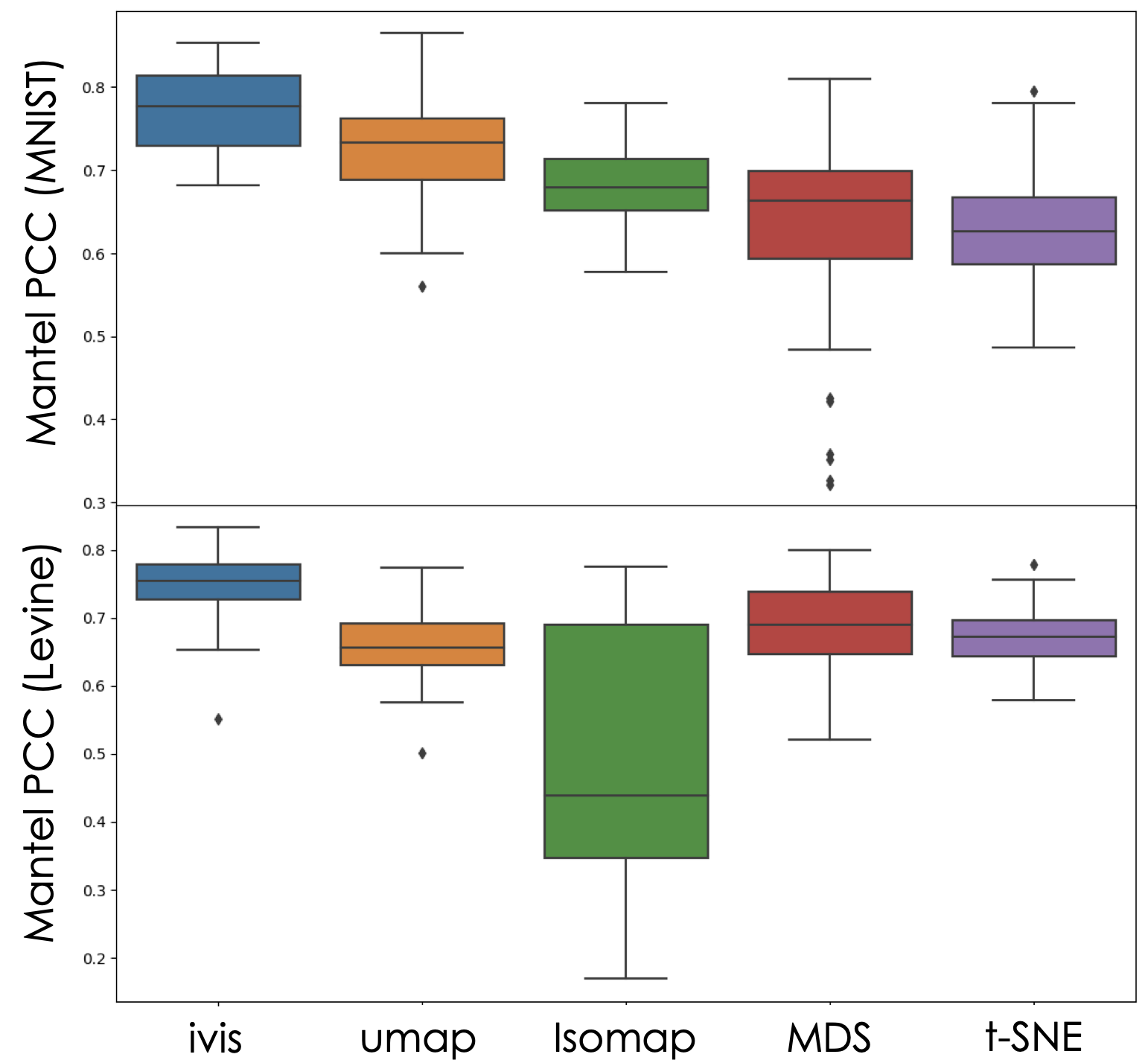# Distance Preservation Benchmarks¶

Dimensionality reduction is crucial for effective manipulation of high-dimensional datasets. However, low-dimensional representations often fail to capture complex global and local relationships in many real-world datasets. Here, we assess how well `ivis` preserves inter-cluster distances in two well-characterised datasets and benchmark performance across several linear and non-linear dimensinality reduction approaches.

## Datasets Selection¶

Two benchmark datasets were used - MNIST database of handwritten digits (70,000 observations, 784 features) and Levine dataset (104,184 observations, 32 features). The Levine dataset was obtained from Data-Driven Phenotypic Dissection of AML Reveals Progenitor-like Cells that Correlate with Prognosis. The 32-dimensional Levine dataset can be downloaded directly from Cytobank.

Both datasets have target `Y` variables. For MNIST, targets take on values [0, 9] and represent hand-written digits, whilst in the Levine dataset targets are manually annotated cell populations [0-13]. Prior to preprocessing, values in both datasets were scaled to [0, 1] range.

• MNIST preprocessing:
```from sklearn.datasets import fetch_openml
from sklearn.preprocessing import MinMaxScaler
X, Y = fetch_openml('mnist_784', version=1, return_X_y=True)
X = MinMaxScaler().fit_transform(X)
```
• Levine preprocessing:
```import pandas as pd
from sklearn.preprocessing import LabelEncoder, MinMaxScaler

data = data.dropna()

features = ['CD45RA', 'CD133', 'CD19', 'CD22', 'CD11b', 'CD4', 'CD8',
'CD34', 'Flt3', 'CD20', 'CXCR4', 'CD235ab', 'CD45', 'CD123',
'CD321', 'CD14', 'CD33', 'CD47', 'CD11c', 'CD7', 'CD15', 'CD16',
'CD44', 'CD38', 'CD13', 'CD3', 'CD61', 'CD117', 'CD49d',
'HLA-DR', 'CD64', 'CD41', 'label']
data = data[features]

X = data.drop(['label'], axis=1).values
X = np.arcsinh(X/5)
X = MinMaxScaler().fit_transform(X)
```

## Accuracy of Low-Dimensional Embeddings¶

To establish how well `ivis` and other dimensionality reduction techniques preserve data structure in low-dimensional space, a Euclidean distance matrix between centroids of the target values in Levine and MNIST datasets was created for the original datasets, respective `ivis` embeddings, as well as UMAP, t-SNE, MDS, and Isomap embeddings. The level of correlation between the original distance matrix and the distance matrices in the embedding spaces was then assessed using the Mantel test. Pearson’s product-moment correlation coefficient (PCC) was used to quantitate concordance between original data and low-dimensional representations. Random stratified subsamples (n=50) of 1000 observations were used to generate a continuum of PCC values for each embedding technique. For all `ivis` runs, only two hyperparameters were set: `k=15` and `model="maaten"`. These are recommended defaults for datasets with <500,000 observations. For other dimensionality reduction methods, default parameters were used.The Mantel Test measures correlation between two distance matrices - embedding space and original space Euclidean distances of cluster centroids. From our experiment, we can conclude that `ivis` preserves inter-cluster distances well, with average PCC being ~0.75 in the MNIST and Levine datasets. Importantly, `ivis` outperformes other dimensionality reduction techniques.